Courses

# Past Year Paper 2018 ( Electrical Engineering)

## 31 Questions MCQ Test | Past Year Paper 2018 ( Electrical Engineering)

Description
This mock test of Past Year Paper 2018 ( Electrical Engineering) for Electrical Engineering (EE) helps you for every Electrical Engineering (EE) entrance exam. This contains 31 Multiple Choice Questions for Electrical Engineering (EE) Past Year Paper 2018 ( Electrical Engineering) (mcq) to study with solutions a complete question bank. The solved questions answers in this Past Year Paper 2018 ( Electrical Engineering) quiz give you a good mix of easy questions and tough questions. Electrical Engineering (EE) students definitely take this Past Year Paper 2018 ( Electrical Engineering) exercise for a better result in the exam. You can find other Past Year Paper 2018 ( Electrical Engineering) extra questions, long questions & short questions for Electrical Engineering (EE) on EduRev as well by searching above.
QUESTION: 1

### For what values of k given below is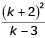an integer?

Solution:

Sincemust be an integer, we can verify the options.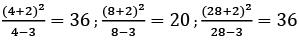So, option (c) Þ 4, 8, 28

QUESTION: 2

### “Since you have gone off the _________, the _________ sand is likely to damage the car”. The words that best fill the blanks in the above sentence are

Solution:

Going off the course – not following the intended route.
Coarse sand – harsh in texture

QUESTION: 3

### The three roots of the equation f (x ) = 0 are x = {-2, 0, 3}. What are the three values of for which f (x - 3)= 0 ?

Solution:

f(x) = 0 for x = {−2,0,3}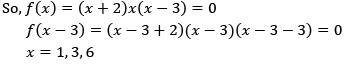QUESTION: 4

Functions, F ( a, b) and G ( a, b) are defined as follows:
F (a, b) = ( a− b)2 and G ( a, b) = |a− b|, where |x| represents the absolute value of x. What would be the value of G ( F (1, 3) , G (1, 3)) ?

Solution: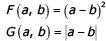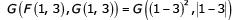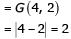QUESTION: 5

“A common misconception among writers is that sentence structure mirrors thought; the more _________ the structure, the ted the ideas”.

Solution:

Because the second half of the sentence illustrates the idea that “structure mirrors thought,” any word that fills the blank must be similar in meaning to “convoluted.” The two words that are similar to “convoluted” are “complicated” and “involved”, which produce sentences alike in meaning. “Fanciful,” while somewhat similar in meaning to “convoluted,” is not as similar to either “complicated” or “involved” as those words are to each other. The other answer choices are not similar in meaning to “convoluted,” and thus do not produce coherent sentences.
Thus the correct answer is complicated.

QUESTION: 6

In a certain code AMCF is written as EQGJ and NKUF is written as ROYJ. How will DHLP be written in the code?

Solution: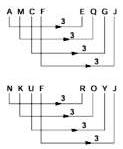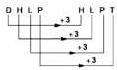QUESTION: 7

An e-mail password must contain three characters. The password has to contain one numeral from 0 to 9, one upper case and one lower case character from the English alphabet. How many distinct passwords are possible?

Solution:

Since, there are three positions which can be filled either by upper case letter, lower case letter or a number from 0 9 −
Hence, total choices are = 26 × 26 × 10 = 6760
3 characters can be arranged in 3! ways & hence total no of password can be = 3! × 6760 = 40560

QUESTION: 8

A designer uses marbles of four different colours of his designs. The cost of each marble is the same, irrespective of the colour. The table below shows the percentage of marbles of each colour used in the current design. The cost of each marble increased by 25%. Therefore, the designer decided to reduce equal numbers of marbles of each colour to keep the total cost unchanged. What is the percentage of blue marbles in the new design?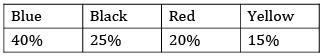Solution:

Assume total number of marbles = 100
Blue marble = 40
Black marble = 25
Red marble = 20
Yellow marble = 15
Cost of 1 marble be Rs. 1
Cost of marbles increased = 25%
New cost of 1 marble = Rs. 1.25
Let x marbles are reduced from each type but the total cost must remain same i.e. Rs 100
Now,
1.25[(40 − x) + (25 − x) + (20 − x) + (15 − x)] = 100
125 − 5x = 100
25 = 5x
x = 5
So, now the numbers of different marbles are
Blue marble = 35
Black marble = 20
Red marble = 15
Yellow marble = 10
% blue marbles in new design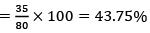QUESTION: 9

P, Q, R and S crossed a lake in a boat that can hold a maximum of two persons, with only one set of oars. The following additional facts are available
i. The boat held two persons on each of the three forward trips across lake and one person on each of the two return trips.
ii. P is unable to row when someone else is in the boat.
iii. Q is unable to row with anyone else except R.
iv. Each person rowed for at least one trip.
v. Only one person can row during a trip.
Who rowed twice?

Solution:

Since, P cannot row with anyone else so P must row while on return journey.
So, on first trip P & S leave in which S rows.
On the return trip P will row
On next trip P will leave with R and R will row & P will get down on other end.
Now R will return & row to pick Q up.
On the last trip Q & R will go in which Q will row the boat.
Hence, R rowed the boat twice

QUESTION: 10

A class of twelve children has two more boys than girls. A group of three children are randomly picked from this class to accompany the teacher on a field trip. What is the probability that the group accompanying the teacher contains more girls than boys?

Solution:

There are 7B and 5G
Through as question is stating 3 students are taken at random.
This can be a possible way to approach it.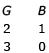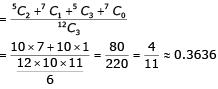QUESTION: 11

Match the transfer functions of the second-order systems with the nature of the system given below.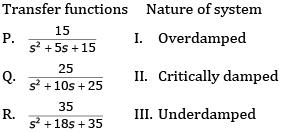Solution: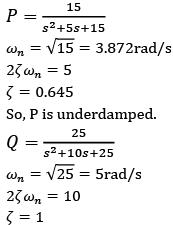So, Q is critically damped.
Observing all the options, option (c) is correct.

QUESTION: 12

Consider a lossy transmission line with V1 and V2 as the sending and receiving end voltages, respectively. Z and X are the series impedance and reactance of the line, respectively. The steady-state stability limit for the transmission line will be

Solution:

With only x :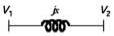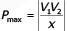With Lossy Tr. Line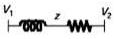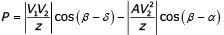∴ With Lossy Line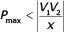QUESTION: 13

Two wattmeter method is used for measurement of power in a balanced three-phase load supplied from a balanced three-phase system. If one of the wattmeter reads half of the other (both positive), then the power factor of the load is

Solution:

In two Wattmeter method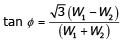Given :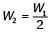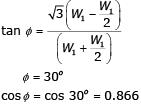QUESTION: 14

A continuous-time input signal x (t ) is an eigen function of an LTI system, if the output is

Solution:

If the output signal is a scalar multiple of input signal, the signal is refereed as an eigen function (or characteristic function) and the multiplier is referred as an eigen value (or characteristic value).
If x(t) is the eigen function and k is the eigen value, then output, y(t) = kx(t).
Hence, the correct option is (A).

QUESTION: 15

In the logic circuit shown in the figure, Y is given by

Solution: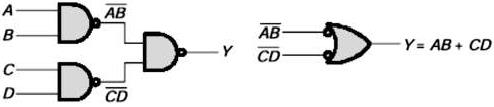*Answer can only contain numeric values
QUESTION: 16

The value of the directional derivative of the function φ( x, y, z ) = xy2 + yz2 + zx2 at the point (2, -1, 1) in the direction of the vector p = i + 2 j +2k is

Solution: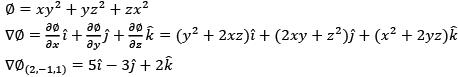The directional derivative of φ ( x, y, z ) at in (2, -1, 1) the direction of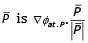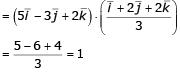*Answer can only contain numeric values
QUESTION: 17

The waveform of the current drawn by a semi-converter from a sinusoidal AC voltage source is shown in the figure. If I0 = 20 A, the rms value of fundamental component of the current is __________ A (up to 2 decimal places).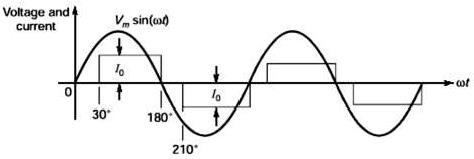Solution: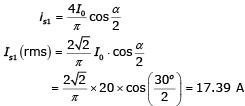*Answer can only contain numeric values
QUESTION: 18

The series impedance matrix of a short three-phase transmission line in phase coordinates is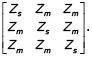If the positive sequence impedance is (1 + j10)Ω, and the zero sequence is (4 + j31)Ω, then the imaginary part of Zm (in W) is __________ (up to 2 decimal places).

Solution: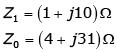We know,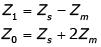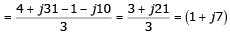The imaginary part of Zm is 7.00.

*Answer can only contain numeric values
QUESTION: 19

In the two-port network shown, the h11 parameter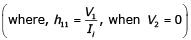in ohms is ________ (up to 2 decimal places).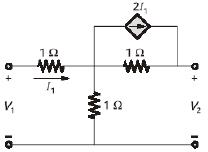Solution:

To find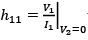the equivalent circuit is given below.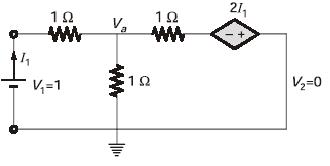By KCL,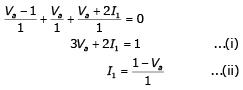Substitute equation (ii) in equation (i),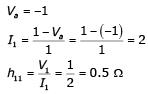QUESTION: 20

The graph of a network has 8 nodes and 5 independent loops. The number of branches of the graph is

Solution: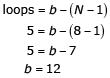QUESTION: 21

In a salient pole synchronous motor, the developed reluctance torque attains the maximum value when the load angle in electrical degree is

Solution:

Reluctance power is given by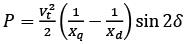(Vt is the line voltage)
So, reluctance torque isSo, torque is maximum when 2δ = 90°
δ = 45°

QUESTION: 22

A single-phase fully controlled rectifier is supplying a load with an anti-parallel diode as shown in the figure. All switches and diodes are ideal. Which one of the following is true for instantaneous load voltage and current?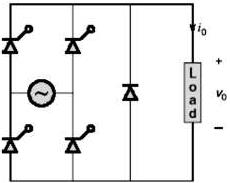Solution:

Since, this converter has freewheeling diode, the output voltage cannot go negative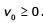Since, diodes and SCR are unidirectional current devices so current cannot reverse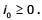*Answer can only contain numeric values
QUESTION: 23

Let f be a real valued function of a real variable defined as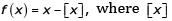denotes the largest integer less than or equal to x. The value of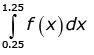is ________ (up to 2 decimal places).

Solution: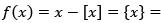fractional part of x Graph of fractional part of x is given below.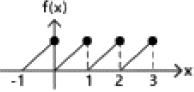It is periodic with period 1.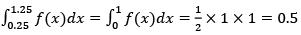QUESTION: 24

A positive charge of 1 nC is placed at (0, 0, 0.2) where all dimensions are in meters. Consider the x-y plane to be a conducting ground plane. Take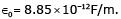The Z component of the E field at (0, 0, 0.1) is closed to

Solution:

Due to infinite conducting plane, we have to consider an image charge symmetrically below plane. Since one charge is placed at (0, 0, 0.2), the image charge will be at (0, 0, -0.2)
Electric field at P will be sum of Field due to Q & -Q Net electric field at point due to a charge Q is given by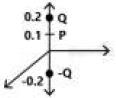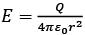So, electric field a point P is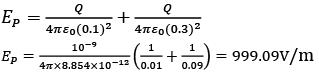Since, it is in downward direction so, Ep = −999.09V/m

*Answer can only contain numeric values
QUESTION: 25

Consider a unity feedback system with forward transfer function given by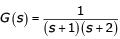The steady-state error in the output of the system for a unit-step input is __________ (upto 2 decimal places).

Solution:

Steady-state error for type-0 and step input,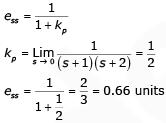*Answer can only contain numeric values
QUESTION: 26

Consider a non-singular 2 * 2 square matrix A. If trace ( A) = 4 and trace ( A2 ) = 5, the determinant of the matrix A is ________ (up 1 decimal place).

Solution:

A is 2 * 2 matrix
Let λ1 and λ2 be the eigen value of matrix A.
Since, sum of eigen values = trace of a matrix
Therefore, λ1 + λ2 = 4
And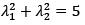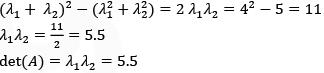*Answer can only contain numeric values
QUESTION: 27

A 1000 * 1000 bus admittance matrix for an electric power system has 8000 non-zero elements. The minimum number of branches (transmission lines and transformers) in this system are ________ (upto 2 decimal places).

Solution:

Number of buses = Number of diagonal elements = 1000
No. of non-zero elements = 8000
No. of non-zero off diagonal elements = 7000
No. of transmission lines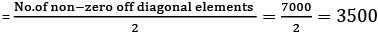QUESTION: 28

In the figure, the voltages are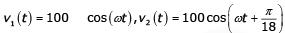and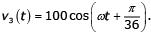The circuit is in sinusoidal steady-state, and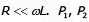and P3 are the average power outputs. Which one of the following statements is true?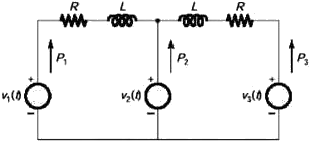Solution: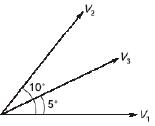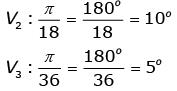So, current will flow from V2 towards V1 and V3.
Hence,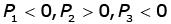QUESTION: 29

The op-amp shown in the figure is ideal. The input impedance is given by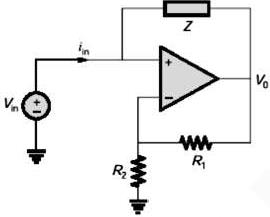Solution:

According to virtual ground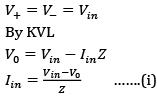Also by voltage division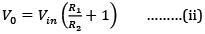Equation (ii) in equation (i)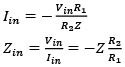QUESTION: 30

The value of the integral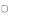in counter clockwise direction around a circle C of radius 1 with center at the point z =−2 is

Solution: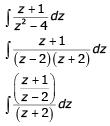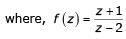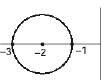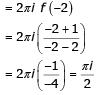*Answer can only contain numeric values
QUESTION: 31

The positive, negative and zero sequence impedances of a 125 MVA, three-phase, 15.5 kV, star-grounded, 50 Hz generator are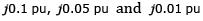respectively on the machine rating base. The machine is unloaded and working at the rated terminal voltage. If the grounding impedance of the generator is j0.01 pu then the magnitude of fault current for a b-phase to ground fault (in kA) is _______ (upto 2 decimal places).

Solution:

For LG fault,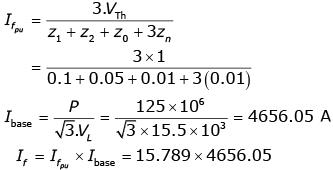= 73516.538 = 73.52kA